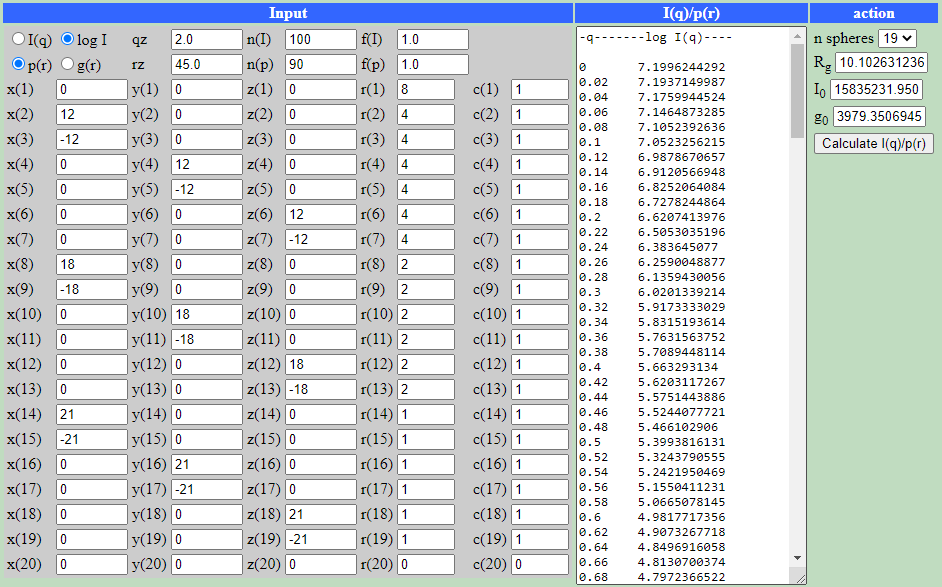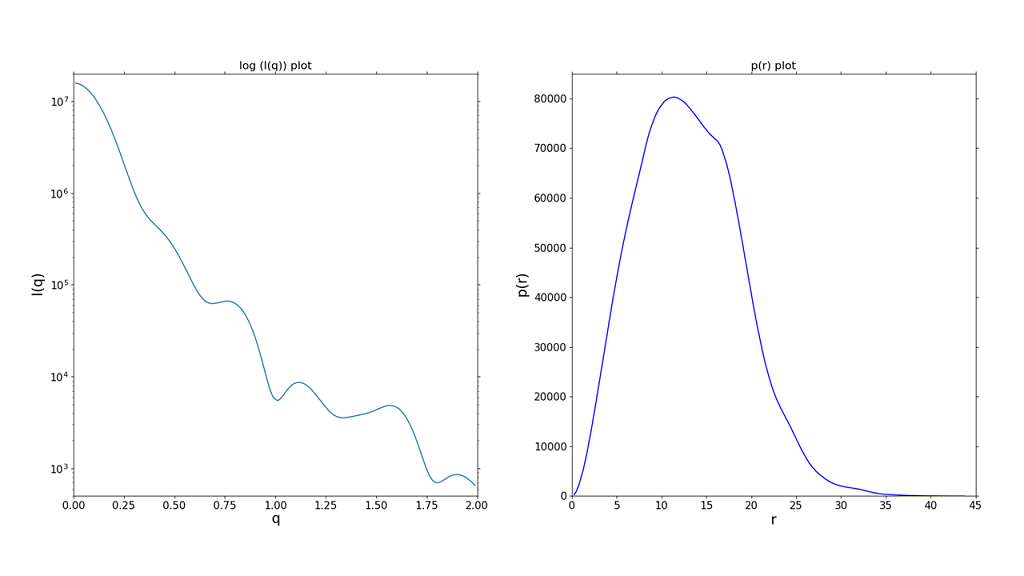## Analytical Calculation of the Scattering Curve I(q) and the Real Space Function p(r) of a Simple Model (Example).

As an example, the scattering curve in reciprocal space and p(r)-function in real space from a model consisting of differently sized spheres are calculated analytically from the Debye-formula in reciprocal space (Debye, P. J. W. (1915), Ann. Phys. 351, 809–823) and its analogue in real-space (Glatter, O. (1980), Acta Phys. Austriaca 52, 243–256). We build a model consisting of 19 spheres (with different radii and constant electron densities) placed on x-y-z coordinates. These coordinates, their respective radii and (optionally different) electron densities are entered as input in the I(q)-p(r)-Debye calculator.The results - I(q) scattering curve and p(r) real-space function of the model (maximum dimension is 44 units) - are shown below:An animation of the used model can be seen hereAuthor: M.Kriechbaum, TU-Graz (2020), e-mail: manfred.kriechbaum@tugraz.at